# Why Randomized Algorithms?

In this post, I want to answer a simple question: how can randomness help in solving a deterministic (non-random) problem?

Let’s start by defining some terminology. An algorithm is just a precisely defined procedure to solve a problem. For example, one algorithm to compute the integral of a function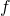on the interval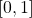is to pick 100 equispaced points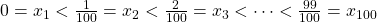on this interval and output the Riemann sum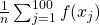. A randomized algorithm is simply an algorithm which uses, in some form, randomly chosen quantities. For instance, a randomized algorithm1This randomized algorithm is an example of the strategy of Monte Carlo integration, which has proved particularly effective at computing integrals of functions on high-dimensional spaces. for computing integrals would be to pick 100 random points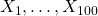uniformly distributed in the intervaland output the average. To help to distinguish, an algorithm which does not use randomness is called a deterministic algorithm.

To address the premise implicit in our central question, there are problems where randomized algorithms provably outperform the best possible deterministic algorithms.2Examples abound in online decision making, distributed computation, linear algebra in the matrix-vector query model, and in simple computational models like single tape Turing machines. Additionally, even for problems for which randomized algorithms have not been formally proven to outperform deterministic ones, the best randomized algorithms we know for many problems dramatically outperform the best-known deterministic algorithms. For example, testing whether an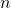-digit number is prime takes roughly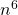operations using the best-known deterministic algorithm and only roughlyoperations allowing randomness. The power of randomness extends beyond discrete problems typically considered by computer scientists to continuous problems of interest to computational mathematicians and scientists. For instance, the (asymptotically) fastest known algorithms to solve Laplacian linear system of equations use random sampling as a key ingredient. The workhorse optimization routines in machine learning are mostly variants of stochastic gradient descent, a randomized algorithm. To list off a few more, randomized algorithms have proven incredibly effective in solving eigenvalue and singular value problems, approximating the trace of a very large matrix, computing low-rank approximations, evaluating integrals, and simulating subgrid phenomena in fluid problems.

This may seem puzzling and even paradoxical. There is no randomness in, say, a system of linear equations, so why should introducing randomness help solve such a problem? It can seem very unintuitive that leaving a decision in an algorithm up to chance would be better than making an informed (and non-random) choice. Why do randomized algorithms work so well; what is the randomness actually buying us?

A partial answer to this question is that it can be very costly to choose the best possible option at runtime for an algorithm. Often, a random choice is “good enough”.

## A Case Study: Quicksort

Consider the example of quicksort. Given a list of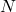integers to sort in increasing order, quicksort works by selecting an element to be the pivot and then divides the elements of the list into groups larger and smaller than the pivot. One then recurses on the two groups, ending up with a sorted list.

The best choice of pivot is the median of the list, so one might naturally think that one should use the median as the pivot for quicksort. The problem with this reasoning is that finding the median is time-consuming; even using the fastest possible median-finding algorithm,3There is an algorithm guaranteed to find the median in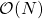time, which is asymptotically fast as this problem could possibly be solved since any median-finding algorithm must in general look at the entire list.quicksort with exact median pivot selection isn’t very quick. However, one can show quicksort with a pivot selected (uniformly) at random achieves the same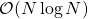expected runtime as quicksort with optimal pivot selection and is much faster in practice.4In fact,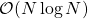is the fastest possible runtime for any comparison sorting algorithm.

But perhaps we have given up on fast deterministic pivot selection in quicksort too soon. What if we just pick the first entry of the list as the pivot? This strategy usually works fairly well too, but it runs into an unfortunate shortfall: if one feeds in a sorted list, the first-element pivot selection is terrible, resulting in a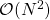algorithm. If one feeds in a list in a random order, the first-element pivot selection has aexpected runtime,5Another way of implementing randomized quicksort is to first randomize the list and then always pick the first entry as the pivot. This is fully equivalent to using quicksort with random pivot selection in the given ordering. Note that randomizing the order of the list before its sorted is still a randomized algorithm because, naturally, randomness is needed to randomize order. but for certain specific orderings (in this case, e.g., the sorted ordering) the runtime is a disappointing. The first-element pivot selection is particularly bad since its nemesis ordering is the common-in-practice already-sorted ordering. But other simple deterministic pivot selections are equally bad. If one selects, for instance, the pivot to be the entry in the position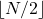, then we can still come up with an ordering of the input list that makes the algorithm run in time. And because the algorithm is deterministic, it runs intime every time with such-ordered inputs. If the lists we need to sort in our code just happen to be bad for our deterministic pivot selection strategy, quicksort will be slow every time.

We typically analyze algorithms based on their worst-case performance. And this can often be unfair to algorithms. Some algorithms work excellently in practice but are horribly slow on manufactured examples which never occur in “real life”.6The simplex algorithm is an example of algorithm which is often highly effective in practice, but can take a huge amount of time on some pathological cases. But average-case analysis of algorithms, where one measures the average performance of an algorithm over a distribution of inputs, can be equally misleading. If one were swayed by the average-case analysis of the previous section for quicksort with first-element pivot selection, one would say that this should be an effective pivot selection in practice. But sorting an already-sorted or nearly-sorted list occurs all the time in practice: how many times do programmers read in a sorted list from a data source and sort it again just to be safe? In real life, we don’t encounter random inputs to our algorithms: we encounter a very specific distribution of inputs specific to the application we use the algorithm in. An algorithm with excellent average-case runtime analysis is of little use to me if it is consistently and extremely slow every time I run it on my input data on the problem I’m working on. This discussion isn’t meant to disparage average-case analysis (indeed, very often the “random input” model is not too far off), but to explain why worst-case guarantees for algorithms can still be important.

To summarize, often we have a situation where we have an algorithm which makes some choice (e.g. pivot selection). A particular choice usually works well for a randomly chosen input, but can be stymied by certain specific inputs. However, we don’t get to pick which inputs we receive, and it is fully possible the user will always enter inputs which are bad for our algorithms. Here is where randomized algorithms come in. Since we are unable to randomize the input, we instead randomize the algorithm.

A randomized algorithm can be seen as a random selection from a collection of deterministic algorithms. Each individual deterministic algorithm may be confounded by an input, but most algorithms in the collection will do well on any given input. Thus, by picking a random algorithm from our collection, the probability of poor algorithmic performance is small. And if we run our randomized algorithm on an input and it happens to do poorly by chance, if we run it again it is unlikely to do so; there isn’t a specific input we can create which consistently confounds our randomized algorithm.

## The Game Theory Framework

Let’s think about algorithm design as a game. The game has two players: you and an opponent. The game is played by solving a certain algorithmic problem, and the game has a score which quantifies how effectively the problem was solved. For example, the score might be the runtime of the algorithm, the amount of space required, or the error of a computed quantity. For simplicity of discussion, let’s use runtime as an example for this discussion. You have to pay your opponent a dollar for every second of runtime, so you want to have the runtime be as low as possible.7In this scenario, we consider algorithms which always produce the correct answer to the problem, and it is only a matter of how long it takes to do so. In the field of algorithms, randomized algorithms of this type are referred to as Las Vegas algorithms. Algorithms which can also give the wrong answer with some (hopefully small) probability are referred to as Monte Carlo algorithms.

Each player makes one move. Your move is to present an algorithm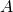which solves the problem. Your opponent’s move is to provide an input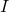to your algorithm. Your algorithmis then run on your opponents inputand you pay your opponent a dollar for every second of runtime.

This setup casts algorithm design as a two-person, zero-sum game. It is a feature of such games that a player’s optimal strategy is often mixed, picking a move at random subject to some probability distribution over all possible moves.

To see why this is the case, let’s consider a classic and very simple game: rock paper scissors. If I use a deterministic strategy, then I am forced to pick one choice, say rock, and throw it every time. But then my savvy opponent could pick paper and be assured victory. By randomizing my strategy and selecting between rock, paper, and scissors (uniformly) at random, I improve my odds to winning a third of the time, losing a third of the time, and drawing a third of the time. Further, there is no way my opponent can improve their luck by adopting a different strategy. This strategy is referred to as minimax optimal: among all (mixed) strategies I could adopt, this strategy has the best performance over all strategies provided my opponent always counters my strategy with the best response strategy they can find.

Algorithm design is totally analogous. For the pivot selection problem, if I pick a the fixed strategy of choosing the first entry to be the pivot (analogous to always picking rock), then my opponent could always give me a sorted list (analogous to always picking paper) and I would always lose. My randomizing my pivot selection, my opponent could input the list in whatever order they choose and my pivot selection will always have the excellent (expected)runtime characteristic of quicksort. In fact, randomized pivot selection is the minimax optimal pivot selection strategy (assuming pivot selection is non-adaptive in that we don’t choose the pivot based on the values in the list).8This is not to say that quicksort with randomized pivot selection is necessarily the minimax optimal sorting algorithm, but to say that once we have fixed quicksort as our sorting algorithm, randomized pivot selection is the minimax optimal non-adaptive pivot selection strategy for quicksort.

## How Much Does Randomness Buy?

Hopefully, now, the utility of randomness is less opaque. Randomization, in effect, allows an algorithm designer to trade algorithm which runs fast for most inputs all of the time for an algorithm which runs fast for all inputs most of the time. They do this by introducing randomized decision-making to hedge against particular bad inputs which could confound their algorithm.

Randomness, of course, is not a panacea. Randomness does not allow us to solve every algorithmic problem arbitrarily fast. But how can we quantify this? Are there algorithmic speed limits for computational problems for which no algorithm, randomized or not, can exceed?

The game theoretic framework for randomized algorithms can shed light on this question. Let us return to the framing of last section where you choose an algorithm, your opponent chooses an input, and you pay your opponent a dollar for every second of runtime. Since this cost depends on the algorithm and the input, we can denote the cost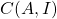.

Suppose you devise a randomized algorithm for this task, which can be interpreted as selecting an algorithmfrom a probability distribution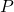over the class of all algorithms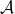which solve the problem. (Less formally, we assign each possible algorithm a probability of picking it and pick one subject to these probabilities.) Once your opponent sees your randomized algorithm (equivalently, the distribution), they can come up with the on-average slowest possible input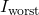and present it to your algorithm.

But now let’s switch places to see things from your opponent. Suppose they choose their own strategy of randomly selecting an inputfrom among all inputs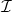with some distribution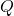. Once you see the distribution, you can come up with the best possible deterministic algorithm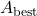to counter their strategy.

The next part gets a bit algebraic. Suppose now we apply your randomized algorithm against your opponents strategy. Then, your randomized algorithm could only take longer on average thanbecause, by construction,is the fastest possible algorithm against the input distribution. Symbolically, we have

(1)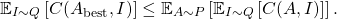Here,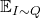and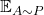denote the average (expected) value of a random quantity when an inputis drawn randomly with distributionor an algorithmis drawn randomly with distribution. But we know thatis the slowest input for our randomized algorithm, so, on average, our randomized algorithm will take longer on worst-case inputthen a random input from. In symbols,

(2)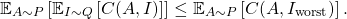Combining Eqs. (1) and (2) gives Yao’s minimax principle9Note that Yao’s minimax principle is a consequence of von Neumann’s minimax theorem in game theory.:

(3)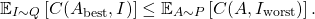In words, the average performance of any randomized algorithm on its worst-case input can be no better than the average performance of the best possible deterministic algorithm for a distribution of inputs.10In fact, there is a strengthening of Yao’s minimax principle. That Eqs. (1) and (2) are equalities whenandare taken to be the minimax optimal randomized algorithm and input distribution, respectively. Specifically, assuming the cost is a natural number and we restrict to a finite class of potential inputs,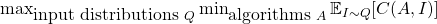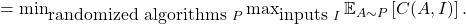This nontrivial fact is a consequence of the full power of the von Neumann’s minimax theorem, which itself is a consequence of strong duality in linear programming. My thanks go to Professor Leonard Schulman for teaching me this result.

This, in effect, allows the algorithm analyst seeking to prove “no randomized algorithm can do better than this” to trade randomness in the algorithm to randomness in the input in their analysis. This is a great benefit because randomness in an algorithm can be used in arbitrarily complicated ways, whereas random inputs can be much easier to understand. To prove any randomized algorithm takes at least cost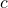to solve a problem, the algorithm analyst can find a distributionon which every deterministic algorithm takes at least cost. Note that Yao’s minimax principle is an analytical tool, not an algorithmic tool. Yao’s principle establishes speed limits on the best possible randomized algorithm: it does not imply that one can just use deterministic algorithms and assume or make the input to be random.

There is a fundamental question concerning the power of randomized algorithms not answered by Yao’s principle that is worth considering: how much better can randomized algorithms be than deterministic ones? Could infeasible problems for deterministic computations be solved tractably by randomized algorithms?

Questions such as these are considered in the field of computational complexity theory. In this context, one can think of a problem as tractably solvable if it can be solved in polynomial time—that is, in an amount of time proportional to a polynomial function of the size of the input. Very roughly, we call the class of all such problems to be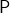. If one in addition allows randomness, we call this class of problems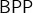.11More precisely,is the class of problems solvable in polynomial time by a Monte Carlo randomized algorithm. The class of problems solvable in polynomial time by a Las Vegas randomized algorithm, which we’ve focused on for the duration of this article, is a possibly smaller class called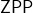.

It has widely been conjectured that: all problems that can be tractably solved with randomness can tractably be solved without. There is some convincing evidence for this belief. Thus, provided this conjecture turns out to be true, randomness can give us reductions in operation counts by a polynomial amount in the input size for problems already in, but they cannot efficiently solve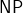-hard computational problems like the traveling salesman problem.12Assuming the also quite believable conjecture that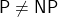.

So let’s return to the question which is also this article’s title: why randomized algorithms? Because randomized algorithms are often faster. Why, intuitively, is this the case? Randomization can us to upgrade simple algorithms that are great for most inputs to randomized algorithms which are great most of the time for all inputs. How much can randomization buy? A randomized algorithm on its worst input can be no better than a deterministic algorithm on a worst-case distribution. Assuming a widely believed and theoretically supported, but not yet proven, conjecture, randomness can’t make intractable problems into tractable ones. Still, there is great empirical evidence that randomness can be an immensely effective algorithm design tool in practice, including computational math and science problems like trace estimation and solving Laplacian linear systems.

## 2 thoughts on “Why Randomized Algorithms?”

1. Sid says:

This was an exciting and informative read!

2. Michael says:

Loved the game theory! Great explanation I learned a lot!